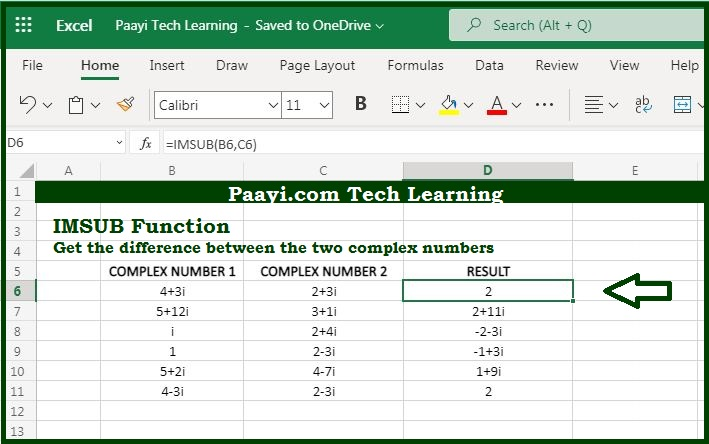# Learn How to Use Microsoft Excel IMSUB Function

Written by | 0 Comments | 686 Views

In this article, you will learn how to use the Microsoft Excel IMSUB function and its prime function in Microsoft Excel. You will also get to know the Microsoft Excel IMSUB function return value and syntax with the help of some examples.

## Microsoft Excel IMSUB Function

The prime function of the Microsoft Excel IMSUB function is to get the difference between the two complex numbers. So, with the help of IMSUB function you can able to return the difference between two complex numbers. The complex number should be in the form of  "x + yi" or "x + yj". You can also use the COMPLEX function to create complex numbers.

### Return Value of IMSUB Function

The return value will be the difference between the complex numbers as a text.

### Syntax of IMSUB Function

=IMSUB(inumber1, inumber2)

Where the arguments:

• inumber1: It is complex number 1.
• inumber2: It is the complex number 2

## How to Use Microsoft Excel IMSUB Function?As we know the IMSUB function you can able to return the difference between the complex numbers. The complex number is input in the form text as,  "x + yi" or "x + yj".